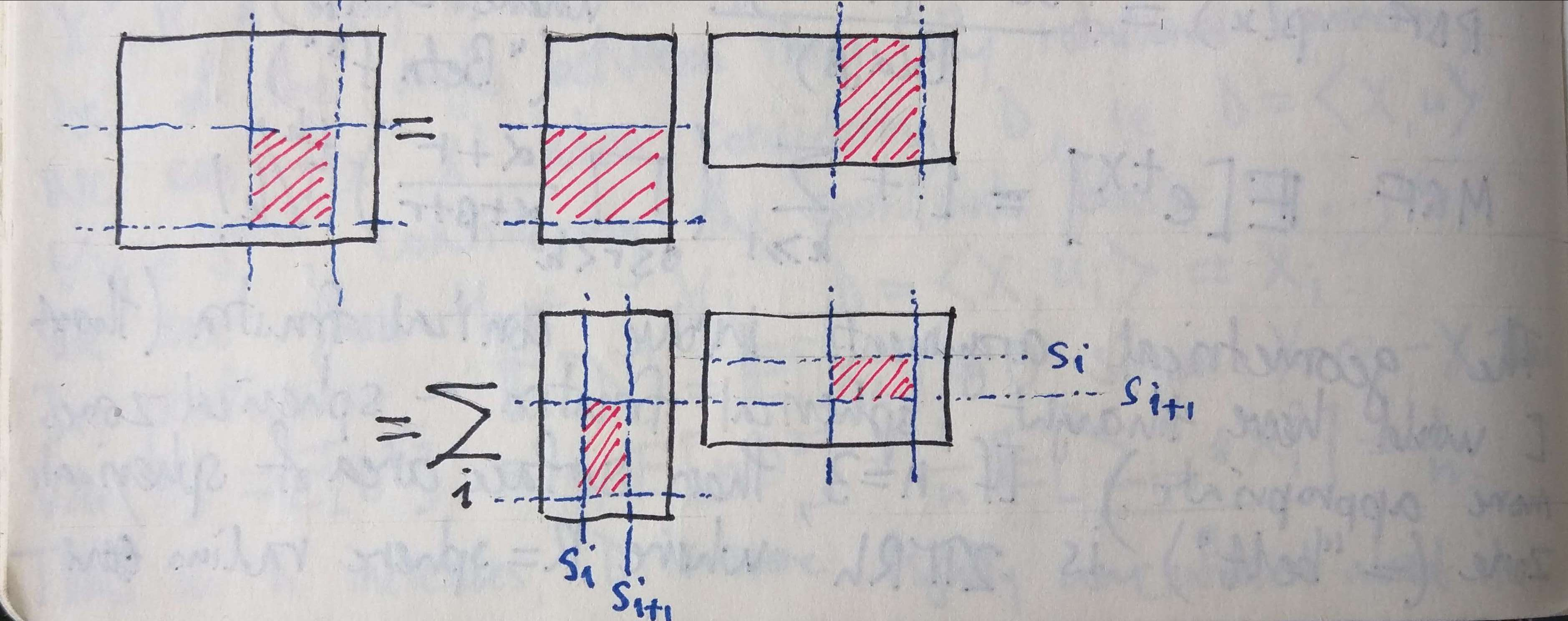# Block Multiplication of Matrices

(We needed this to derive the conditional distribution of a multivariate Gaussian).

Consider a matrix product. Partition the two outer dimensions (i.e. the rows ofand the columns of) and the one inner dimension (i.e. the columns ofand the rows of) arbitrarily. This defines a “block decomposition” of the productand of the factorssuch that the blocks ofare related to the blocks ofandvia the familiar formula for components of the product, i.e..

Pictorially, we have the following:Arithmetically, this is easy to prove by considering the formula above for the components of the product. The partitioning of the outer dimensions comes for free, while the partitioning of the inner dimension just corresponds to partitioning the summation:.

Zooming out to a categorical level, we can see that there is nothing peculiar about this situation. If, in an additive category, we have three objectswith biproduct decompositions, and a chain of morphisms:then this “block decomposition of matrices” finds expression as a formula inusing the injection and projection morphisms associated with each biproduct factor.